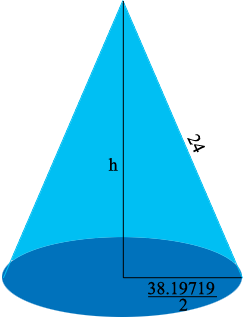SEARCH HOMEMath Central Quandaries & QueriesQuestion from Charles: Sheet metal cone. I need a cone with a finished base of 38.19719 diameter The cone is to be from a 48 diameter round with the wedge cut out. The best calculation I have is the arc is 286.479. (correct?) Could you verify this arc angle but more so what is the cone height?Hi Charles,

I agree with your calculation. The wedge to be removed sits on an arc of length 286.479 (inches?). You didn't include units.When you roll up the wedge to form a conethe slant height of the cone is the radius of the "round" and the radius of the circular base is half its diameter. I let the height be $h$ inches and then the triangle in the diagram is a right triangle so Pythagoras Theorem gives you

$h^2 + \left( \frac{38.19719}{2} \right)^2 = 24^2 .$

Thus

$h = \sqrt{24^2 - \left( \frac{38.19719}{2} \right)^2} = 14.534 \mbox{ inches.}$

HarleyMath Central is supported by the University of Regina and The Pacific Institute for the Mathematical Sciences.